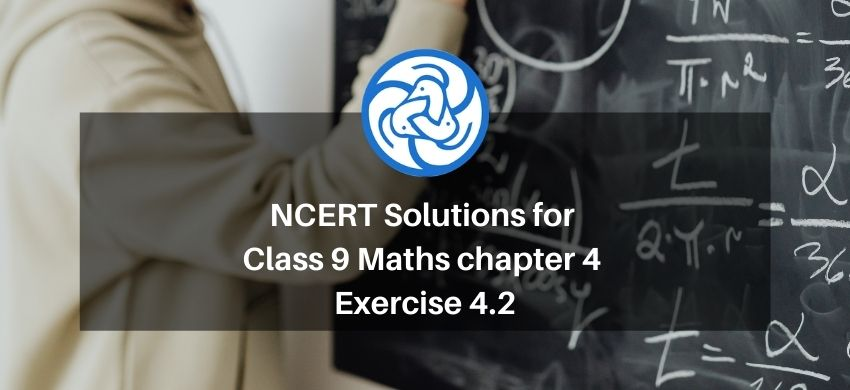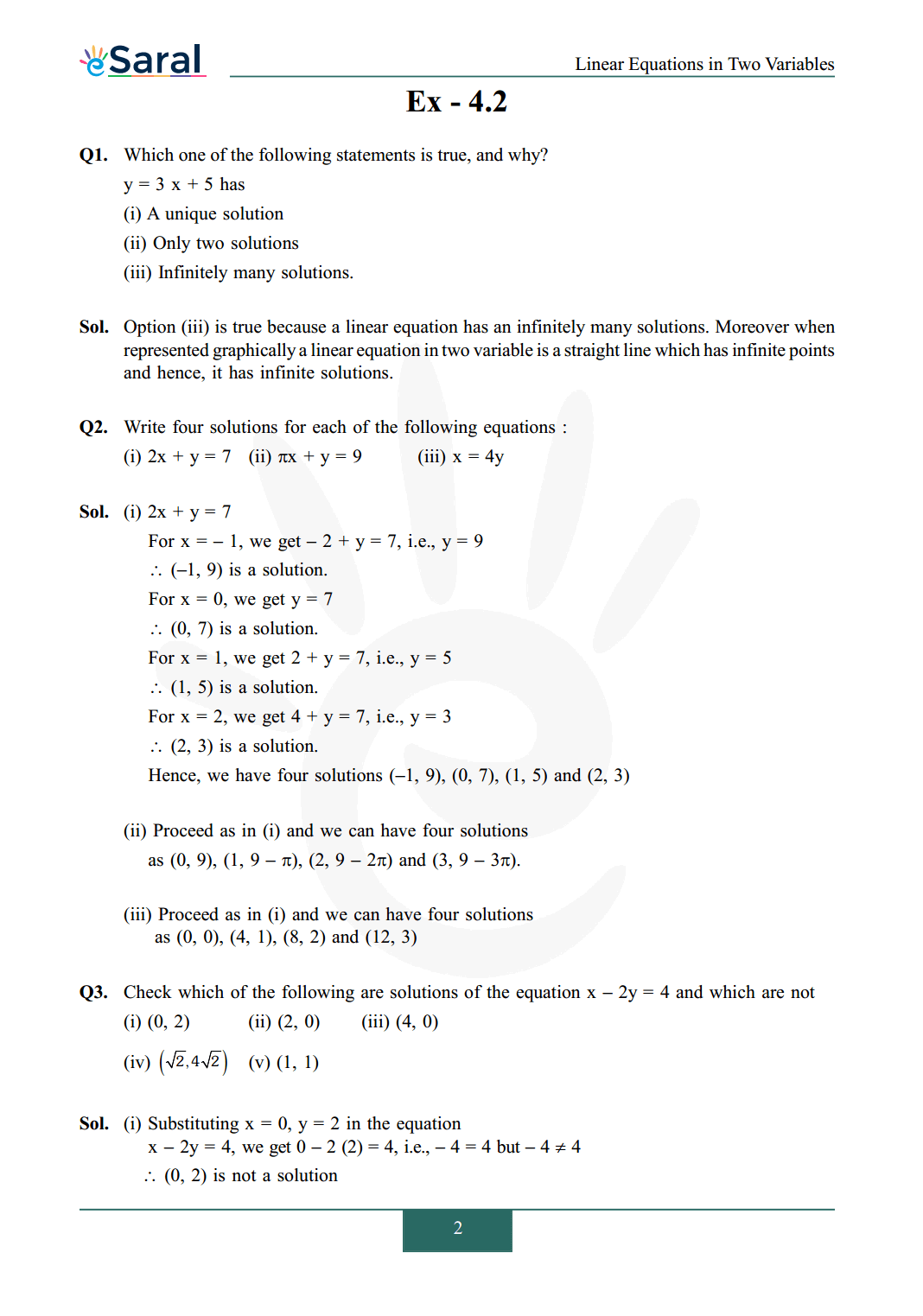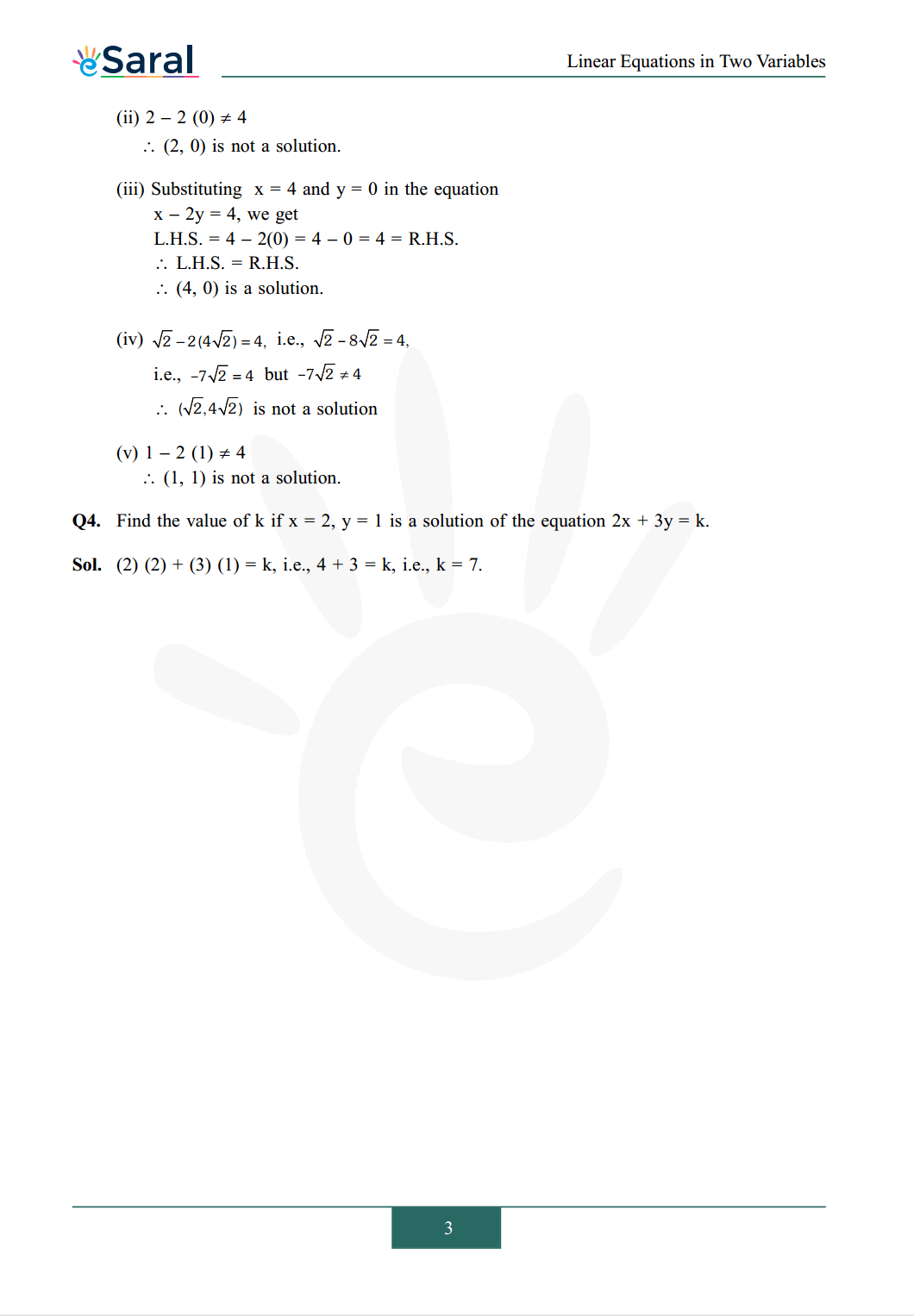Download the eSaral app and start learning from Kota's top IITians and doctors.

# NCERT Solutions for Class 9 Maths chapter 4 Exercise 4.2 - Linear Equations in Two VariablesHey, are you a class 9 Student and Looking for Ways to Download NCERT Solutions for Class 9 Maths chapter 4 Exercise 4.2? If Yes then you are at the right place.

Here we have listed Class 9 maths chapter 4 exercise 4.2 solutions in PDF that is prepared by Kota’s top IITian’s Faculties by keeping Simplicity in mind.

If you want to score high in your class 9 Maths Exam then it is very important for you to have a good knowledge of all the important topics, so to learn and practice those topics you can use eSaral NCERT Solutions.

So, without wasting more time Let’s start.

### Download The PDF of NCERT Solutions for Class 9 Maths chapter 4 Exercise 4.2 "Linear Equations in Two Variables"#### All Questions of Chapter 4 Exercise 4.2

Once you complete the chapter 4 then you can revise Ex. 4.2 by solving following questions

Q1. Which one of the following statements is true, and why?
$\mathrm{y}=3 \mathrm{x}+5$ has
(i) A unique solution
(ii) Only two solutions
(iii) Infinitely many solutions.

Q2. Write four solutions for each of the following equations:
(i) $2 \mathrm{x}+\mathrm{y}=7$
(ii) $\pi \mathrm{x}+\mathrm{y}=9$
(iii) $\mathrm{x}=4 \mathrm{y}$

Q3. Check which of the following are solutions of the equation $\mathrm{x}-2 \mathrm{y}=4$ and which are not
(i) $(0,2)$
(ii) $(2,0)$
(iii) $(4,0)$
(iv) $(\sqrt{2}, 4 \sqrt{2})$
(v) $(1,1)$

Q4. Find the value of $\mathrm{k}$ if $\mathrm{x}=2, \mathrm{y}=1$ is a solution of the equation $2 \mathrm{x}+3 \mathrm{y}=\mathrm{k}$.

Class 9 Maths Chapter 3 Exercise 3.1 Solutions PDF

Class 9 Maths Chapter 3 Exercise 3.2 Solutions PDF

Class 9 Maths Chapter 3 Exercise 3.3 Solutions PDF

If you have any Confusion related to NCERT Solutions for Class 9 Maths chapter 4 Exercise 4.2 then feel free to ask in the comments section down below.

To watch Free Learning Videos on Class 9 by Kota’s top Faculties Install the eSaral App